# PSAT Math : Decimals

## Example Questions

← Previous 1 3 4 5

### Example Question #2 : How To Find The Decimal Equivalent Of A Fraction

How much less is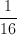than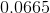?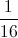is greater than.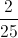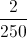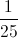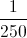Explanation: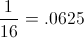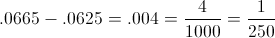### Example Question #3 : How To Find The Decimal Equivalent Of A Fraction

The ogre under the bridge eatsof a pizza and then throws the rest of the pizza to the rats. The rats eatof what is left. What fraction of the pizza is left when the rats are done?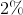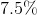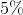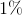Explanation:

1/5 of the pizza is left after the ogre eats his share. The rats eat 3/4 of that, so 1/4 of 1/5 of the pizza is left.

1/4 * 1/5 = 1/20 = 5%

### Example Question #1 : Decimals

Express as a decimal: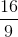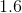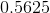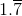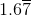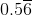Explanation:

Divide 16 by 9: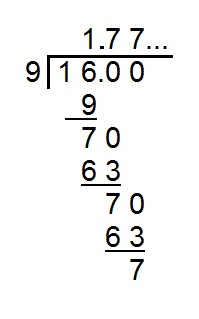The "7" repeats forever, so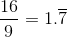### Example Question #2 : Decimals

Express as a decimal: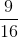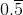Explanation:

Divide 9 by 16: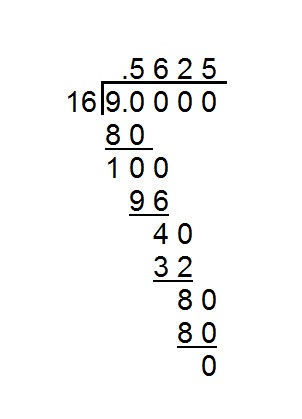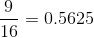### Example Question #3 : Decimals

Convert the fraction to a decimal.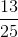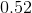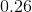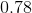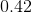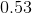Explanation:

We can multiply our numerator and denominator by, which leads to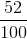. From here we divide by our denominator, moving the decimal two places to the left.

### Example Question #1 : Decimals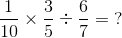0.10

0.01

0.07

0.04

0.05

0.07

Explanation:

Multiply numerator by the other numerator and multiply the denominator by the other denominator for multiplication. To divide fractions, switch numerator and denominator and treat it as multiplication. The answer is 0.07.

### Example Question #2 : How To Find The Fractional Equivalent Of A Decimal

Write 0.45 as a fraction.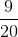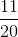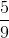Explanation:

.45 is equivalent to 45 out of 100, or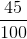.

Divide both the numerator and denominator by 5 to simplify the fraction:### Example Question #4 : Decimals

Express as a fraction: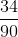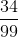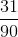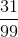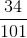Explanation:

Let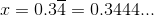Then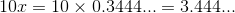and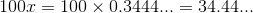Subtract: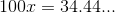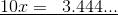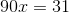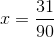### Example Question #1 : How To Multiply Decimals

45.728 x 3.2 = ?

14632.96

146.3296

14.63296

146329.6

1463.296

146.3296

Explanation:

Multiply the numbers out in long format, then move the decimal point over the total number of decimal points in the two numbers (3 in the first, 1 in the second, so 4 total):

45.728 * 3.2 = 91456

91456 + 1371840 = 1,463,296 → 146.3296

Or just count the decimal points in the answer and make sure it's 4.

### Example Question #2 : How To Multiply Decimals

At the farmer's market, oranges are $0.30 each, apples are$0.25 each, bananas are $0.40 each, and tomatoes are$0.60 each. If Scott buys 3 oranges, 7 apples, 4 bananas, and 8 tomatoes, how much does he spend?

$9.05$7.75

$6.80$10.40

$11.40 Correct answer:$9.05

Explanation:

To find out how much Scott spends, we need to multiply the quantity of each fruit by its price and add them all up.

3 * $0.30 + 7 *$0.25 + 4 * $0.40 + 8 *$0.60 = \$9.05

← Previous 1 3 4 5